Latest Banking jobs   »   Quantitative Aptitude Quiz For SBI Clerk...

# Quantitative Aptitude Quiz For SBI Clerk Prelims 2021- 23rd May

Directions (1-6): The line graph given below shows the number of stationaries sold by two shops X and Y on five different days of a week. Stationary includes pens, books and copies only.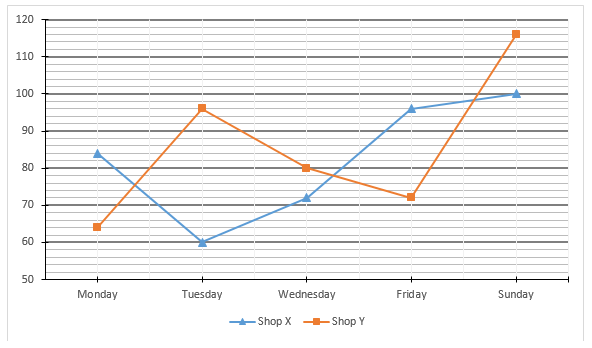Q1. Total number of stationaries sold by shop X on Monday and Wednesday together is how much more/less than the total number of stationaries sold by shop Y on Tuesday and Friday together?
(a) 10
(b) 12
(c) 14
(d) 16
(e) 18

Q2. What is the ratio of the total number of stationaries sold by both shop on Tuesday to that on Wednesday?
(a) 39 : 38
(b) 38 : 39
(c) 41 : 38
(d) 37 : 35
(e) None of these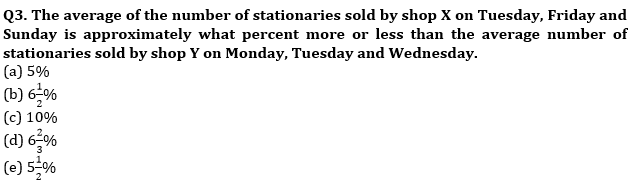Q4. Total number of stationaries sold on Sunday by both shop is what percent of the total number of stationaries sold on Monday and Wednesday together by both shop.
(a) 65%
(b) 60%
(c) 80%
(d) 75%
(e) 72%

Q5. Out of the total number of stationaries sold by shop Y on Monday, Tuesday and Wednesday together, 16 ¼ % are the copies and the ratio between number of books sold to that of pen sold is 1:2. Find the difference between the number of pens sold and number of copies sold.
(a) 95
(b) 96
(c) 93
(d) 92
(e) 98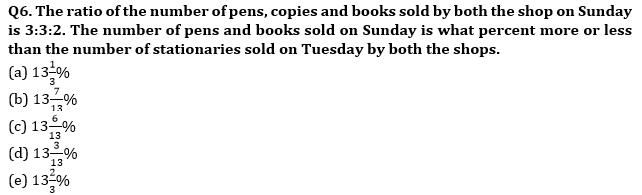Directions (7-9): In the following questions two quantities are given for each question. Compare the numeric value of both the quantities and answers accordingly.
Q7.
“Quantity I. 12x² – 61x + 77 =0
Quantity II. 20x² – 91x + 99 =0
(a) Quantity I > Quantity II
(b) Quantity II > Quantity I
(c) Quantity I ≥ Quantity II
(d) Quantity II ≤ Quantity I
(e) Quantity I = Quantity II or relation can’t be established.

Q8. Quantity I. The average of the five consecutive odd numbers is 23. Then, find the difference between the square of largest and smallest odd numbers.
Quantity II. Find the value of x (Use approximation)
495.01 + 63.98% of 949.963 = 738.0 + x
(a) Quantity I > Quantity II
(b) Quantity II > Quantity I
(c) Quantity I ≥ Quantity II
(d) Quantity II≤ Quantity I
(e) Quantity I = Quantity II or relation can’t be established.

Q9. Quantity I. 10x² – 9x + 2 = 0
Quantity II. 15x² – 11x + 2 =0
(a) Quantity I > Quantity II
(b) Quantity II > Quantity I
(c) Quantity I ≥ Quantity II
(d) Quantity II ≥ Quantity I
(e) Quantity I = Quantity II or relation can’t be established.

Directions (10-14): Given below is the bar graph which shows the girls percentage out of total students who went to five different places i.e. A, B, C, D & E to spend their summer vacation.
NOTE- Girls percentage is given out of total students who visited a particular place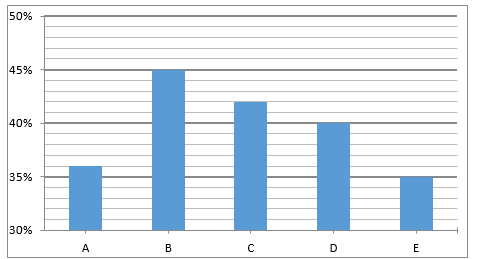Note :- Students= Boys + Girls.

Q10. If difference between total girls who went to A and total girls who went to D was zero then find the ratio of boys who went to D to girls who went to A.
(a) 3 : 5
(b) 4 : 3
(c) 4 : 5
(d) 3 : 2
(e) 3 : 4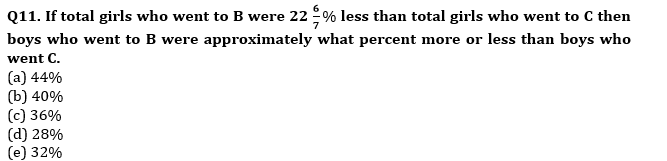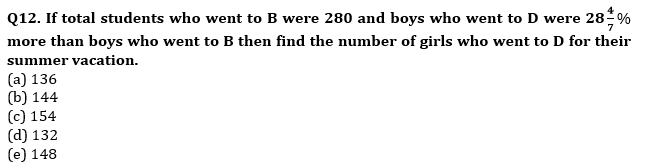Q13. If total number of boys who went to C and E together were 327 and number of boys went E were 73 ⅓% more than number of girls who went C then find average of students who went to C and E for their summer vacation?
(a) 245
(b) 257
(c) 265
(d) 270
(e) 275

Q14. If girls who went to D and E were 160 and 140 respectively then find the difference between number of boys who went D and E.
(a) 10
(b) 20
(c) 30
(d) 40
(e) 50

Directions (15 – 17): Given below in each question there are two statements (I) and (II). You must determine; which statement is enough to give the answer of question. Also, there are five alternatives given, you have to choose one alternative as your answer of the questions:

Q15. What is the time period for which Mr. Jindal invested his capital?
I. Mr. Jindal and his partner Mr. Ravi invest in a partnership with total capital of Rs.20 lacs.
II. Ratio of time period of investment of Mr. Jindal and his partner Mr. Ravi is 4 : 5 and profit sharing ratio of Mr. Jindal and his partner Mr. Ravi is 12 : 25.
(a) Only statement I is sufficient
(b) Only statement II is sufficient
(c) Statement I and II both together sufficient
(d) Either statement I or Statement II alone sufficient
(e) Neither statement I nor statement II sufficient

Q16. A bag contains ‘a’ blue ball, ‘b’ green balls and 6 red balls. Find difference between blue and green balls.
I. If one ball is taken out randomly from bag, probability of being its blue is4/15.
II. If one ball is taken out randomly from bag, probability of being its green is 1/3.
(a) Only statement I is sufficient
(b) Only statement II is sufficient
(c) Statement I and II both together sufficient
(d) Either statement I or Statement II alone sufficient
(e) Neither statement I nor statement II sufficient

Q17.In how much time a boatman can reach a point and come back which is 120km away from his house?
I. He can row 40km upstream in same time in which he can row 80km downstream
II. Time taken by boatman to row 60km upstream is 3.75 hours more than time taken by him to row same distance in downstream.
(a) Only statement I is sufficient
(b) Only statement II is sufficient
(c) Statement I and II both together sufficient
(d) Either statement I or Statement II alone sufficient
(e) Neither statement I nor statement II sufficient

Directions (18-20): What will come in place of question mark (?) in the following questions?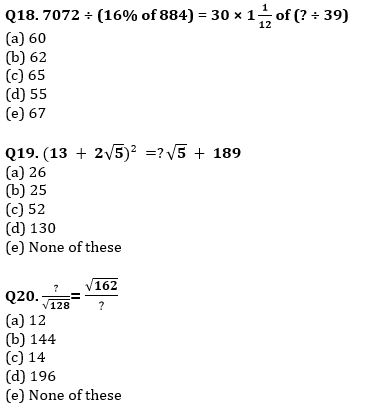Practice More Questions of Quantitative Aptitude for Competitive Exams:

###### SBI Clerk Study Plan 2021

Solutions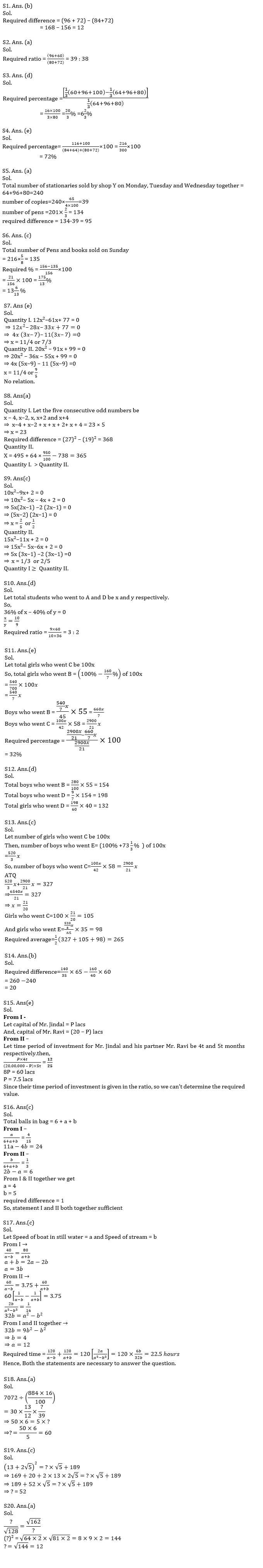#### Congratulations!Incorrect details? Fill the form again here

•Quantitative Aptitude Quiz For IBPS RRB ...
•Quantitative Aptitude Quiz For IBPS Cler...
•Quantitative Aptitude Quiz For IDBI AM/E...
•Quantitative Aptitude Quiz For IDBI AM/E...
•Reasoning Ability Quiz For IDBI 2022- 2n...
•Reasoning Ability Quiz For SBI Clerk Pre...